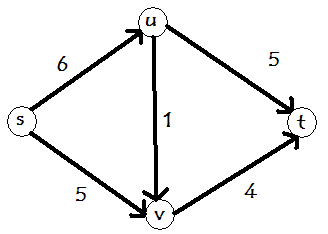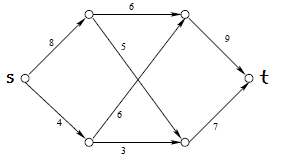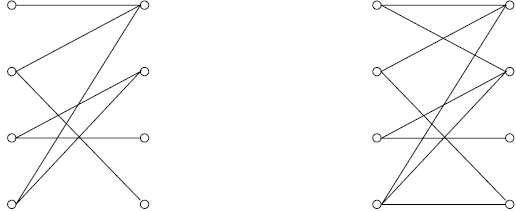# The Hot Shot

In this episode, Charlie suggests using directed network flows to analize the murdered women's routine. Let us first review the needed definitions.

## Directed Network Flow

A directed network flow is a directed graph with the following conditions:

1. There are two special points, usually denoted by s (source) and t (target). s has no edges coming into it, and t has no edges going out of it
2. Every edge e in the directed graph has been assigned a non-negative number c(e), called capacity. This number gives the maximum amount of flow that the edge can carry.
A flow is a function from the edges to the positive numbers satisfying:
1. For any edge e, f(e) is at least 0 and at most c(e).
2. For any edge e, the amount of flow entering e is the same as the amount of flow going out of e.### Network flows as distribution systems

In real life, directed network flows are used to model distribution systems, as follows: Suppose Company Y delivers TV to XAL-MART. For this,  Company Y has a distribution network that looks like directed graph above, where s represents the storage facility, t represents XAL-MART, and u and v are intermediate facilities. The edge capacities represent the maximum number of TV sets that can be sent between two points. For instance, the edge (u,v) has capacity 1, and so facility u is able to send one TV set to v. What is the maximum number of TV sets that Company Y can deliver to XAL-MART? What would the flow be?

Suppose you have to get some amount of material X from the distribution center s to a retailer located at point t. The directed edges represent the routes between different points, and the capacities represent the

Activity 1
• What is the maximum flow that can be carried from s to t in the picuture below?• What if the edge with capacity 5 is reversed?
• Charlie points out that in this case, they only need to look at some chain in the women's routines, represented in a "change of flow". Analyze how the maximum flows you just found changes in the case the edge with capacity 9 is no longer available.

There exists an algorithm to compute the maximum flow of a network known as the Ford-Fulkerson algorithm. The basic idea behind the algorithm is that if there exists a path from s to t that has available capacity, then send some flow along that path. Later improvements consisted in selecting a "good" available path.

### PERFECT MATCHINGS

A very common application of network flows is to perfect matchings. For this, we recall that a bipartite graph is a graph (collection of vertices and edges connecting some of the vertices)  where the vertices can be divided into two sets L and R (which stand for left and right) in such a way that no two vertices in L (or in R) share an edge. The following picture illustrates this conceptwhere all the vertices in the left hand side belong to L and those in the right hand side belong to R.

The maximum matching problem consists of finding a set of edges M as large as possible so that any vertex in the graph is an endpoint of at most one edge in M.

Activity 2 Find the a maximum matching for each of the graphsActivity 3: Finding perfect matchings using directed network flows

The perfect matching problem ask something about an undirected graph. So in order to use directed network flows, we need to construct a directed graph. We proceed as follows: Given a bipartite graph with L and R having the same number of elements, construct a directed network as described below.

(*) Construct two new vertices: one labeled s to the left of L and one labeled t to the right of R.
(*) Construct a directed edge from s to any vertex in L.
(*) Construct a directed edge from any vertex in R to t.
(*)  Direct the edges from the bipartite graph from L to R.
(*) Assign 1 as the capacity to all the directed edges constructed.

1. Draw the directed networks corresponding to the bipartite graphs in Activity 2.
2. What is the maximum flow that can be pushed in each network?
3. Convince yourself that a bipartite graph has a perfect matching if and only if the corresponding directed network can carry a maximum flow with value the number of elements in L (or R)

Activity 4: Assigning Resources

Suppose that you are an assistant at your school's library. Your supervisor has given you the following assignment: There are n students in the need to write a paper on civil wars in Latin America for their history class. Unfortunately, they procrastinated and have only one week write their papers, but the library only owns m books on the topic. Due tho this constraints, you supervisor asked each person to submit a list with with the titles of at most two books. Since the students are picky, any of them who cannot obtain all the books requested, she will ask for an extension. Your job is to allocate the m books to guarantee that the largest number of people are able to obtain the books they requested (and thus, to the teacher's contempt, the number of students asking for an extension is minimum). How can you do this?